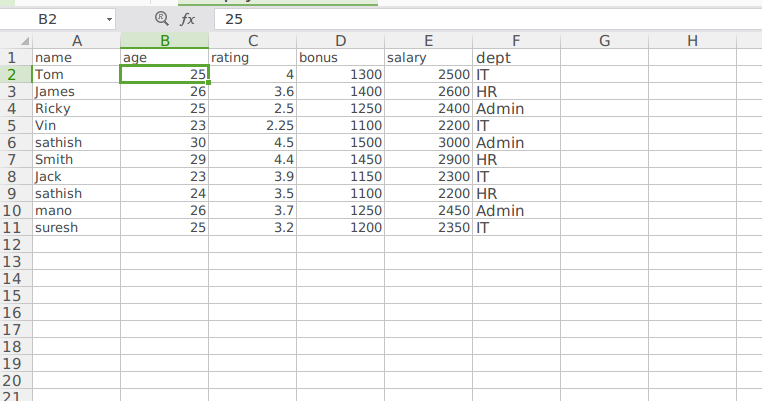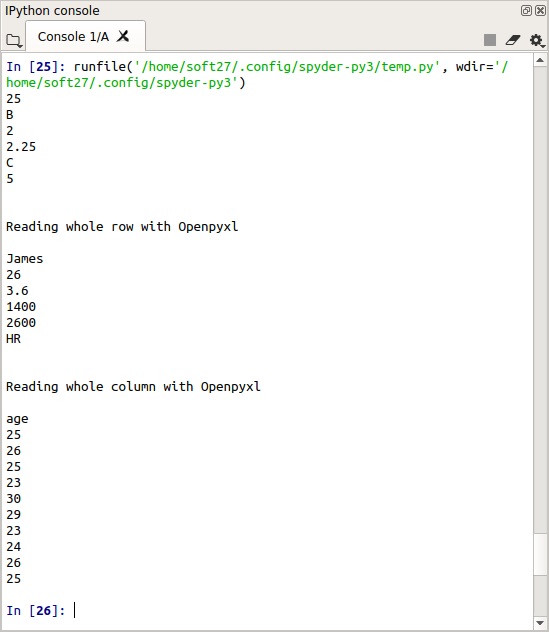• #5, First Floor, 4th Street Dr. Subbarayan Nagar Kodambakkam, Chennai-600 024 Landmark : Samiyar Madam
• pro@slogix.in
• +91- 81240 01111

### How to read data from excel file using Openpyxl in python?

###### Description

To see how to extract data from an excel file using python3.

###### Openpyxl:

The openpyxl module allows Python program to read and modify Excel files.

Its the open source library designed with python.

We can read, write, rename , merge data using this module.

###### Sample Code

#import openpyxl module

import openpyxl

#create workbook object

workbook(‘Employee.xlsx’)

#data extract from sheet1

sheet=(wb[‘Sheet1’])

#take a data from a cell

a=sheet[‘B2’]

print(a.value)

print(a.column)

print(a.row)

b=sheet.cell(row=5,column=3)

print(b.value)

print(b.column)

print(b.row)

print(“\n”)

for c in range(1,7):

d=sheet.cell(row=3,column=c)

print(d.value)

print(“\n”)